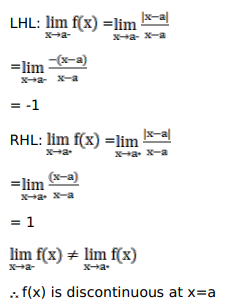# Prove thatQuestion:

Prove that

f(x)=\left\{\begin{aligned} \frac{|x-a|}{x-a}, & \text { when } x \neq a ; \\ 1, & \text { when } x=a \end{aligned}\right. is discontinuous at $x=a$

Solution: